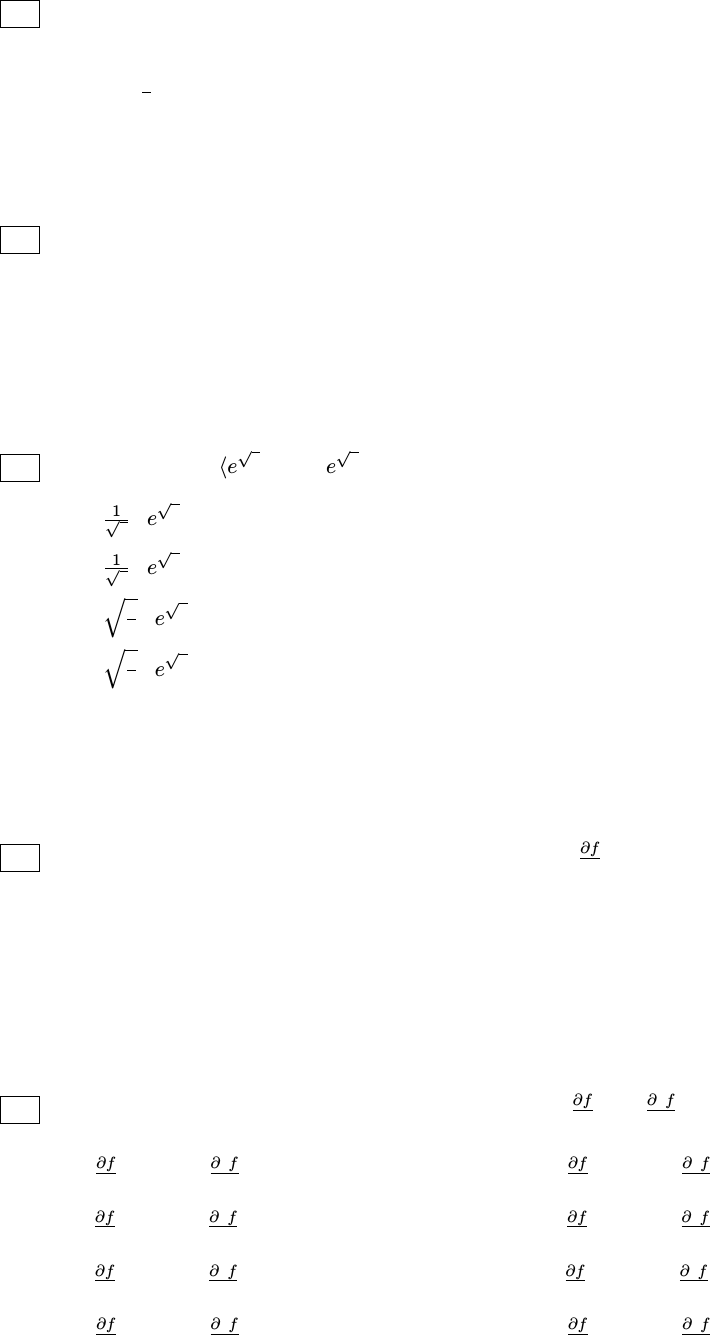Study Guides (400,000)
US (230,000)
Harvard (700)
MATH (400)
MATH 21A (100)
All (100)
Midterm

# MATH 21A Harvard 21a Spring 09Midterm1 Spr07Exam

Department
Mathematics
Course Code
MATH 21A
Professor
All
Study Guide
Midterm

This preview shows half of the first page. to view the full 3 pages of the document.Math 21a Old Exam One – Spring 2007 Spring, 2009
Part I: Multiple choice. Each problem has a unique correct answer. You do not need to justify your
answers in this part of the exam.
1The vectors A=2i+ (t1)j+ 2kand B=j+tkare parallel when:
(a) t= 0;
(b) t=1
3;
(c) t= 1 and t= 2;
(d) all values of t;
(e) no values of t.
2If Aand Bare vectors in space, then the expression (A+B)·(A×B) equals
(a) 0(the zero vector) because A+Band A×Bare always parallel;
(b) 0 (the number zero) because Aand Bare always perpendicular to A×B;
(c) a positive number since (A+B)·(A×B) is the volume of a parallelepiped;
(d) undeﬁned since we cannot take the dot product of a vector and a scalar;
(e) an arbitrary number, depending on the particular vectors Aand B.
3The curve r(t) = he2tcos t, e2tsin ti, 0 tπ, has length
(a) 1
3e2π1;
(b) 1
2e2π1;
(c) q2
3e2π1;
(d) q3
2e2π1;
(e) given by a deﬁnite integral which cannot be evaluated explicitly;
(f) given by an indeﬁnite integral.
The questions in problems 4-5 refer to the marked points in the diagram below, which shows the level
curves of a function f(x, y).
4At which of the labeled points is the partial derivative f
x strictly positive?
(a) at Sand T(b) at Sand W
(c) at Tand W(d) at Vand W
(e) at Vand Y(f) only at V
(g) at Wand Y(h) only at T
5At the point Y, what can one say about the values of f
y and 2f
y2?
(a) f
y >0 and 2f
y2>0 (b) f
y >0 and 2f
y2<0
(c) f
y <0 and 2f
y2>0 (d) f
y <0 and 2f
y2<0
(e) f
y = 0 and 2f
y2>0 (f) f
y = 0 and 2f
y2<0
(g) f
y >0 and 2f
y2= 0 (h) f
y <0 and 2f
y2= 0